# Data classification methods

When you classify your data, you can use one of many standard classification methods provided in ArcGIS Pro, or you can manually define your own custom class ranges. Classification methods are used for classifying numerical fields for graduated symbology.

## Manual interval

Use manual interval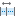to define your own classes, to manually add class breaks and to set class ranges that are appropriate for the data. Alternatively, you can start with one of the standard classifications and make adjustments as needed.

## Defined interval

Use defined interval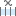to specify an interval size to define a series of classes with the same value range. For example, if the interval size is 75, each class will span 75 units. The number of classes, based on the interval size and maximum sample size, is determined automatically. The interval size must be small enough to fit the minimum number of classes allowed, which is three.

## Equal interval

Use equal interval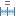to divide the range of attribute values into equal-sized subranges. This allows you to specify the number of intervals, and the class breaks based on the value range are automatically determined. For example, if you specify three classes for a field whose values range from 0 to 300, three classes with ranges of 0–100, 101–200, and 201–300 are created.

Equal interval is best applied to familiar data ranges, such as percentages and temperature. This method emphasizes the amount of an attribute value relative to other values. For example, it shows that a shop is part of the group of shops that make up the top one-third of all sales.

## Quantile

In a quantile classification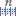, each class contains an equal number of features. A quantile classification is well suited to linearly distributed data. Quantile assigns the same number of data values to each class. There are no empty classes or classes with too few or too many values.

Because features are grouped in equal numbers in each class using quantile classification, the resulting map can often be misleading. Similar features can be placed in adjacent classes, or features with widely different values can be put in the same class. You can minimize this distortion by increasing the number of classes.

## Natural breaks (Jenks)

With natural breaks classification (Jenks)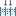, classes are based on natural groupings inherent in the data. Class breaks are created in a way that best groups similar values together and maximizes the differences between classes. The features are divided into classes whose boundaries are set where there are relatively big differences in the data values.

Natural breaks are data-specific classifications and not useful for comparing multiple maps built from different underlying information.

This classification is based on the Jenks Natural Breaks algorithm. For further information, see Univariate classification schemes in Geospatial Analysis—A Comprehensive Guide, 6th edition; 2007–2018; de Smith, Goodchild, Longley.

## Geometrical interval

The geometrical interval classification scheme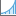creates class breaks based on class intervals that have a geometric series. The geometric coefficient in this classifier can change once (to its inverse) to optimize the class ranges. The algorithm creates geometric intervals by minimizing the sum of squares of the number of elements in each class. This ensures that each class range has approximately the same number of values in each class and that the change between intervals is fairly consistent.

This algorithm was specifically designed to accommodate continuous data. It is a compromise between the equal interval, natural breaks (Jenks), and quantile methods. It creates a balance between highlighting changes in the middle values and the extreme values, thereby producing a result that is visually appealing and cartographically comprehensive.

One example for using the geometrical interval classification is a rainfall dataset in which only 15 out of 100 weather stations (less than 50 percent) have recorded precipitation, and the rest have no recorded precipitation, so their attribute values are zero.

## Standard deviation

The standard deviation classification method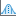shows you how much a feature's attribute value varies from the mean. The mean and standard deviation are calculated automatically. Class breaks are created with equal value ranges that are a proportion of the standard deviation—usually at intervals of one, one-half, one-third, or one-fourth—using mean values and the standard deviations from the mean.